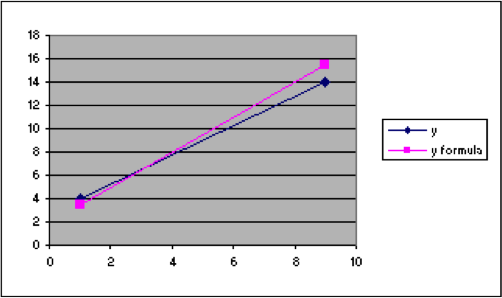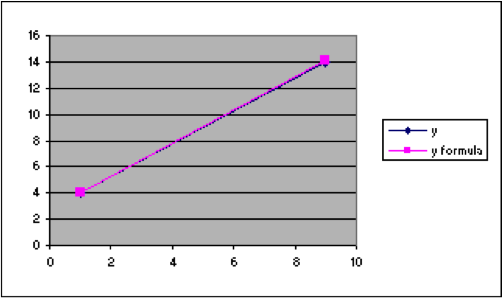## G1.06: Exploring by Graphing Part II

Example 16. Continue with the previous spreadsheet to explore to find an equation of the line through the points (1,9) and (4,14).

1. Starting with the previous spreadsheet, look at the points and guess what the intercept and slope might be.   I guess that the intercept might be 2 and the slope might be 1.5. So put those guesses in Column G.   In G2 put 2 and in H2 put the label “intercept.”       In G3 put 1.5 and in H3 put the label “intercept.”
2. In column C, put the label “y formula” in cell C1. Then, in cell C2, put the formula for a line, , but put it in the appropriate form to use the values for the slope and intercept in column G and the x values in column A.   That is, in cell C2 put =$G$3*A2+$G$2.A B C D E F G H 1 x y y formula 2 1 4 3.5 2 intercept 3 9 14 15.5 1.5 slope 4 5 6 7 8 9 10 11 12 13 14
1. Notice that the line for the formula is close, but not correct. So adjust the values for the slope and intercept until it looks right. First, it looks as if the intercept is actually a little higher than the formula indicates. So try 3 for that.   And then it looks as if the slope is a little lower than the formula indicates.       So try 1.4 for that. That looks better, but still not correct.
2. The intercept of 3 is still too large. Try 2.8.   The slope of 1.4 is still too large. Try 1.3.   This looks better, but it could still be improved upon.
3. Leave the intercept at 2.8 and try a slope of 1.25. That looks pretty good on the graph. The numbers in the formula column show that it is not quite correct, but this is about as good as we’d expect to get from just fitting by eye.   We can use algebra to find more precise values.A B C D E F G H 1 x y y formula 2 1 4 4.05 2.8 intercept 3 9 14 14.05 1.25 slope 4 5 6 7 8 9 10 11 12 13 14 15

Using algebra here, as illustrated in Examples 5, 6, 11, and 12, we find that the equation of the line through the points (1,4) and (9,14) is $y=1.25x+2.75$. The formula we have found just by looking at the graph and approximating is very close to the correct formula.